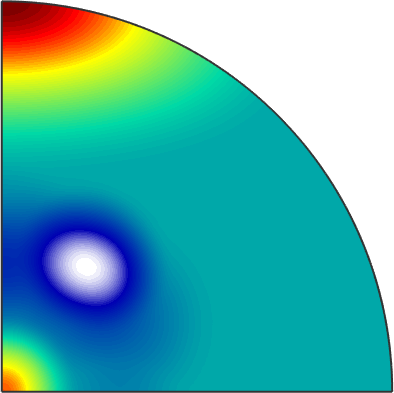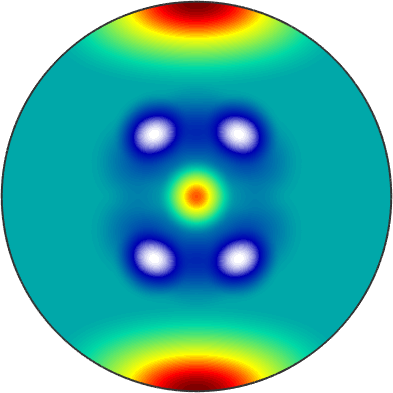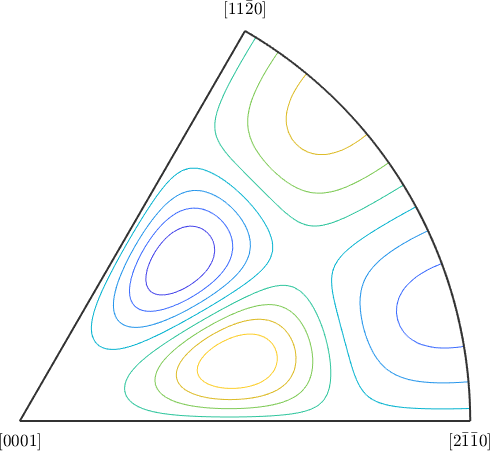S2FunHarmonicSym edit page

S2FunHarmonicSym is an extension of the S2FunHarmonic class which stores the symmetry with the function.

## Defining a S2FunHarmonic

Definition via symmetrisation

The simplest way to define a S2FunHarmonicSym is through the symmetrisation of an ordinary S2FunHarmonic.

• this symmetrises the function and gives back the result with the symmetry attached• Note that only the important part with respect to the symmetry is plotted
• you can plot the full sphere using the argument 'complete'

Definition via function handle

If you have a function handle for the function you could create a S2FunHarmonicSym via quadrature. At first lets define a symmetry and a function handle which takes vector3d as an argument and returns double:

Now you can call the quadrature command to get sFs2 of type S2FunHarmonicSym

## Visualization

The plot commands for a S2FunHarmonicSym by default plot the function only on the fundamenta Sector of the symmetry. E.g. the default plot-command look as followsAnother Example is the contour plot